# Q. 1. Fill in the blank and rewrite the completed statements 8

1. A large amount of is present in the air around us.

Ans A large amount of Heat is present in the air around us.

2. A liquid does not have a definite shape but it has a definite …………… .

Ans A liquid does not have a definite shape but it has a definite Volume.

3. The heat contained in a substance is the measure of the kinetic energy of atoms in the substance.

Ans The heat contained in a substance is the measure of the total kinetic energy of atoms in the substance.

4. The linear expansion of a solid is the increase in length of a wire or a rod of a solid due to increase in its…………… .

Ans The linear expansion of a solid is the increase in length of a wire or a rod of a solid due to increase in its temperature.

4. The hot object gives away heat while the cold object heat.

Ans The hot object gives away heat while the cold object absorbs heat.

5. A thermometer is used to measure ……….

Ans A thermometer is used to measure temperature.

6. The apparatus used to measure heat is called a …………. .

Ans The apparatus used to measure heat is called a calorimeter.

7. Temperature is the measure of the kinetic energy of the atoms in a substance.

Ans Temperature is the measure of the average kinetic energy of the atoms in a substance.

# Q. 2. Find the odd one out 2

1. Celsius, Kelvin, Fahrenheit, Meter

Ans Meter its not an unit for temperature

2. Copper, Iron, Mercury, Gold

Ans Mercury its comes under expansions of liquid rest all are for expansion of solid.

# Q.3. Find co-related terms 5

1. Liquids : volumetric expansion coefficient :: Gases:

Ans Constant pressure expansion coefficient.

Liquids possess only volumetric expansion coefficient. Similarly, gases show expansion in volume only under constant pressure conditions. Hence, they possess only constant pressure expansion coefficient.

2. Heat : joule :: Specific heat :

Ans J/kg °C

Heat is measured in joule in SI system. Similarly, specific heat is measured in J/kg °C in SI system

3. Clinical thermometer : 35 °C to 42 °C :: laboratory thermometer:

Ans 40 °C to 110 °C

Clinical thermometers are used to measure temperatures in the range 35 °C to 42 °C. Similarly, laboratory thermometers used to measure temperatures in the range of 40 °C to 110 °C.

4. Thermometer : temperature :: calorimeter:

Ans Heat content

Thermometer is used to measure temperature. Similarly, calorimeter is used to measure heat content of the object.

5. Heat content: total kinetic energy of atoms :: Temperature:

Ans Average kinetic energy of atoms

Total kinetic energy of atoms is a measure of heat contained in a substance. Similarly, temperature is related to the average kinetic energy of the atoms.

# Q.4. Extra data 3

1. Expansions of gas & Expansions of liquid

###### Ans
 Expansions of gas Expansions of liquid A gas does not even have a fixed volume. A liquid does not have a definite shape but it has a definite volume.

2. Complete the paragraph:

The Sun is the biggest source of heat received by the earth. A large amount of heat is generated due to the…………… taking place in its ……………. In this process …………… fuse together to form ,generating heat in the process. Some of it reaches the earth in the form of …………… .

Ans The Sun is the biggest source of heat received by the earth. A large amount of heat is generated due to the Nuclear fusion taking place in its center. In this process Hydrogen nuclei fuse together to form Helium nuclei, generating heat in the process. Some of it reaches the earth in the form of light and heat.

######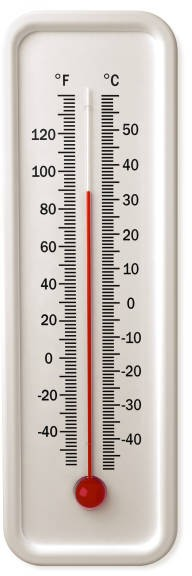3.
 Which effect of heat does the above instrument work on? Ans The figure shows a thermometer which works on the expansion of liquids due to heat.

# Q.5. Match the pair

###### 1. Whom should I pair with?
 Column – A Column – B i. Temperature of healthy human body a. 296 K ii. Boiling point of water b. 98.6 °F c. 212°C
###### Ans
 i. Temperature of healthy human body 98.6 °F ii. Boiling point of water 212°C

2.

Column – B

Column – A

 i. Alcohol a. 1.0 ii. Water b. -38.8 Celsius c. Water
###### Ans
 Column – A Column – B i. Alcohol Water ii. Water 1.0

3. Whom should I pair with ?

 Column – A Column – B i. Room temperature a. 0 C ii. Freezing point of water b. 98.6 F c. 296 K
###### Ans
 i. Room temperature 296 K ii. Freezing point of water 0 C

4.

 Column – A Column – B i. Freezing point of water a. 0.58 ii. Freezing point of mercury b. 32 Fahrenheit c. -38.8 Celsius
###### Ans
 i. Freezing point of water 32 Fahrenheit ii. Freezing point of mercury -38.8 Celsius

# Q. 6. State True or False 11

1. Objects contract on heating.

Ans False – Objects expand on heating.

2. Atoms of a solid are free.

Ans False – Atoms of a solid are bound tightly to each other.

3. The average kinetic energy of atoms in a hot object is less than the average kinetic energy of atoms in a cold object.

Ans False – The average kinetic energy of atoms in a hot object is more than the average kinetic energy of atoms in a cold object.

4. Calorimeter is used to measured heat.

###### Ans True

5. Higher the temperature of the solid, faster is their velocity of oscillation.

###### Ans True

6. A liquid have a definite shape but it does not have definite volume.

Ans False – A liquid does not have a definite shape but it has a definite volume.

7. Joule is the unit of heat.

###### Ans True

8. Different substances have different specific heats.

###### Ans True

9. Heat flows from an object at higher temperature to an object at lower temperature.

###### Ans True

10. Temperature is measured in units of meter.

Ans False – Temperature is measured in units of Celsius.

11. The temperature of a substance is measured in Joules –

Ans False – The Temperature of a substance is measured in C or Kelvin or F, while the heat of the substance is measured in joules.

# Q.7. Name the following 3

1. Name the units in which temperature is measured.

Ans Celsius, Fahrenheit and Kelvin.

2. Gas expands on heating but if the gas is kept in a closed box, its volume cannot increase but its pressure?

Ans Increases

3. Name any two expansions of solid.

Ans i. Volumetric expansion of solids

ii. Areal expansions of solids

# Q. 8. Multiple Choice Questions (Activity) 2

1. Boiling point of mercury is?

a. 100 Celsius          b. 356.7 Celsius

c. 278.9 Celsius        d. 265.8 Celsius

Ans Option b.

2. What is calorimeter made of ?

a. Silver     b. Copper    c. Platinum      d. Steel

Ans Option b.

# Q. 9. Answer in one sentence 3

1. Define expansion of liquid.

Ans Expansion of liquid can be define as a liquid which does not have a definite shape but it has a definite volume.

2. A bridge is made from 20 m long iron rods. At temperature 18 0C, the distance between two rods is 0.4 cm. Up to what temperature will the bridge be in good shape?

Ans 35.4 Celsius

3. Give equation showing the relations between the three units of temperature

###### Ans

(F−32) / 9  = C / 5

K = C + 273.15

# Q. 10. Multiple Choice Questions (Experiment) 2

1. A large amount of heat is generated due to …………… .

a. Nuclear Fusion        b. Sun         c. Chemical energy         d. Earth

Ans Option a.

2 . △T is the change in …………… .

a. Constant pressure          b. Initial volume

c. Temperature                   d. Final volume

Ans Option c.

# Q. 11. Solve Numerical problems: 8

1. Considering the reading shown on the instrument in °F, determine its exact value in °C using the relation between Fahrenheit and Celsius scale.

Ans Given : Temperature in Fahrenheit (F) = 100 °F To find: Temperature in Celsius (C)

Formula:

F − 32/9 = C/5

Calculation  : From formula,

100 − 32/9 = C/5

∴ C = 68 × 5/ 9 = 37.78 °C

The value of the reading in °C is 37.78 °C.

2. When a substance having mass 3 kg receives 600 cal of heat, its temperature increases by 10 °C. What is the specific heat of the substance?

Ans Given : Mass of the substance (m) = 3 kg =1 × 103 g,

amount of heat given (Q) = 600 cal,

inital temperature (T1) = 10 °C,

final temperature (T2) = 70 °C

To find : Specific heat of substance (c)

Formula : Q = m × c × ΔT

Calculation : From formula,

= 600 × 3 × 103 × c × (70 – 10)

= 3 × 103 × c × 60

∴ c = 600 / 60 × 3 × 103 =10/3 x10 -3

###### = 0.0033 cal/g °C

The specific heat of the substance is 0.0033 cal/g °C

3. Two substances A and B have specific heats c and 2 c respectively. If A and B are given Q and 4Q amounts of heat respectively, the change in their temperatures is the same. If the mass of A is m, what is the mass of B?

Ans Given : Specific heat of substance A (cA) = c,

specific heat of substance B (cB) = 2c,

amount of heat given to A (QA) = Q,

amount of heat given to B (QB) = 4Q,

ΔTA = ΔTB, mass of substance A (mA) = m

To find : Mass of substance B (mB)

Formula : Q = m × c × ΔT

Calculation : From formula, for substance A,

QA = mA × cA × ΔTA ………………….i

For substance B,

QB = mB × cB × ΔTB ………………………ii

Dividing equation (ii) by equation (i), we get,

QB/QA = mB/mA × cB/cA × ΔTB/ ΔTA

Substituting given data,

4Q/Q =  mB/m×  2c/c

mB / m = 4/2 =2

∴ mB = 2m

The mass of substance B is 2m.

4. At 15 °C the height of Eifel tower is 324 m. If it is made of iron, what will be the increase in length in cm, at 30 °C?

Ans Given :   Initial height of Eiffel tower (I1) = 324 m,

initial temperature (T1) =   15 °C,

final temperature (T2) = 30°C,

Coefficient of linear expansion for iron (λ iron) = 11.5 × 10-6 (1/°C)

To find : Increase in the height (I2 – I1) Formula : I2 = I1 (1 + λ ΔT)

Calculation : From formula,

I2 = I1 + λ I1 ΔT

∴ I2 = I1 + λ I1 ΔT

= 11.5 × 10-6 × 324 × (30 – 15)

= 0.056m

###### = 5.6 cm

The increase in the height of the Eiffel tower will be 5.6 cm.

# Q. 12. Write Short Notes 4

1. Note on specific heat.

Ans i. The specific heat of an object is the amount of heat required to increase the temperature of unit mass of that substance through one degree.

ii. This is represented by the symbol ‘c’.

iii. Different substances have different specific heats.

iv. Its unit in SI is Joule /(kg 0C) and in CGS is cal /( gm 0C)

2. Write note on expansion

Ans i. When heat is given to any substance, its temperature increases and it expands

ii. Its expansion depends on the increase in its temperature

iii. Solids, liquids and gases, all expand on receiving heat

# Q. 13. Distinguish between 4

1. Thermometer and Calorimeter.

###### Ans
 THERMOMETER CALORIMETER i. Thermometer is used to measure the temperature of an object i. A calorimeter is used to measure the heat content of an object ii. iii. It has a narrow glass tube which has a bulb at one end ii. Calorimeter has two vessels, an inner and an outer one It is usually made of glass. iii. It is made up of copper Thermometer id not thermally isolated from the surroundings The calorimeter is thermally isolated from the surroundings iv. iv.

2. What is the difference between heat and temperature? What are their units?

###### Ans
 Heat Temperature i. The heat contained in a substance is the total kinetic energy of the atoms in the substance. i. The temperature is measure of the average kinetic energy of the atoms in the substance. ii. Heat content of any substance depends on the number of atoms present in it. ii. Temperature of a substance does not depend on the number of atoms present in it. iii. It is measured using calorimeter. iii. It is measured using thermometer. iv. Heat is measured in joules (J) in SI system while, in calories (cal) in CGS system. iv. The units of temperature are Celsius ( C), Fahrenheit ( F) and Kelvin (K).

# Q. 14. Activity based question (3 mks) 3

###### 1
 Substances Specific heat Cal/(gm Celsius) Substances Specific heat cal/(gm Celsius) Aluminium …………… Iron ……………
 Hydrogen …………… Copper …………… Water …………… Mercury ……………

Ans

 Substances Specific heat Cal/(gm Celsius) Substances Specific heat cal/(gm Celsius) Aluminium 0.21 Iron 0.11 Hydrogen 0.58 Copper 0.09 Water 0.03 Mercury 0.03

# Q. 15. Activity based questions.(2 mks) 6

1. Label different types of thermometers.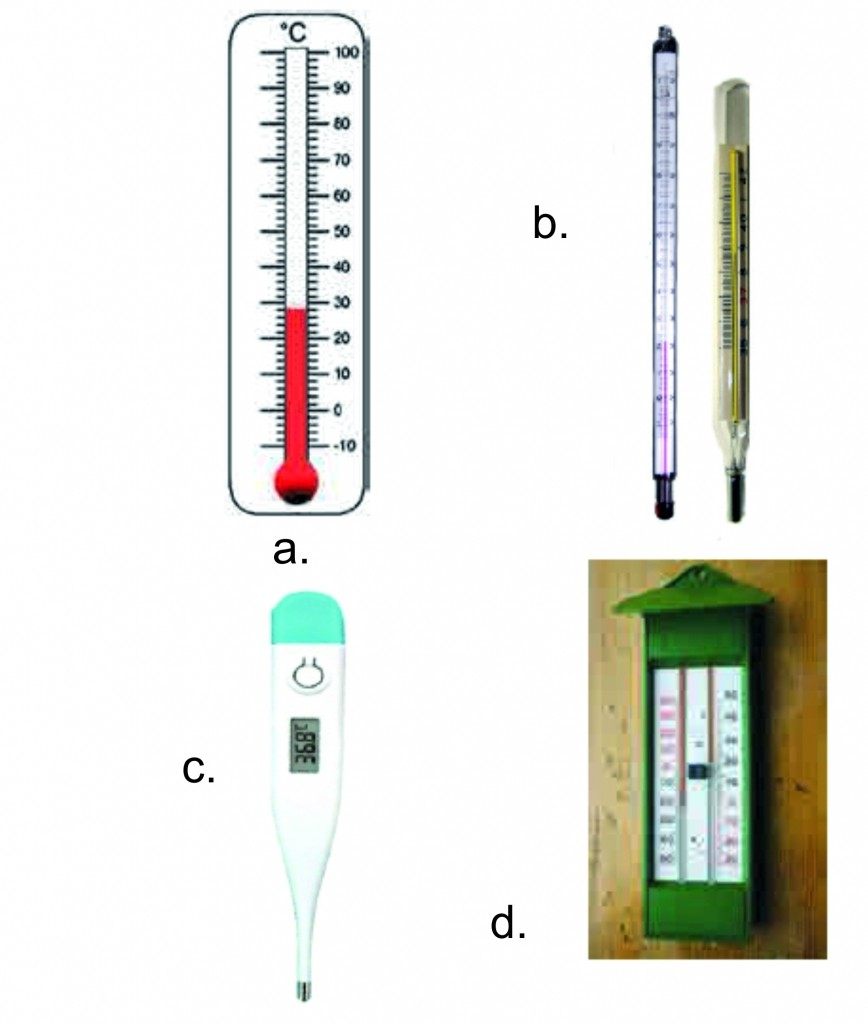Ans  :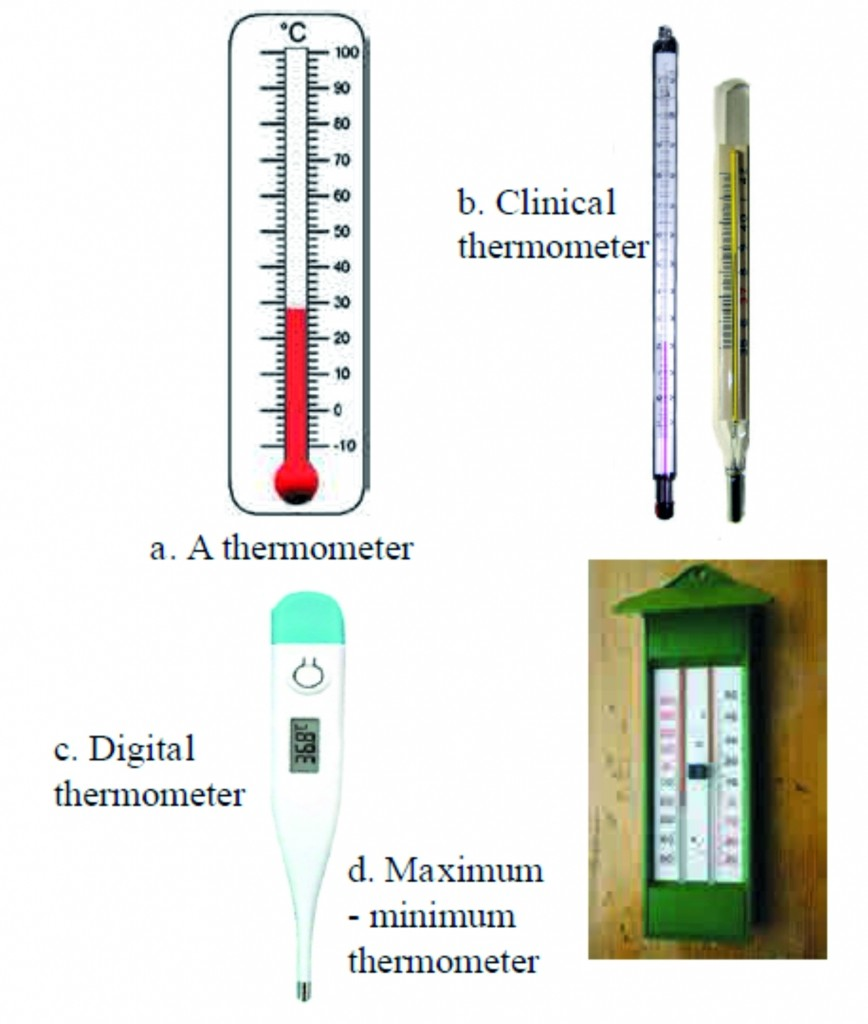###### 2. Label the diagram of effect of heat on a gas###### Ans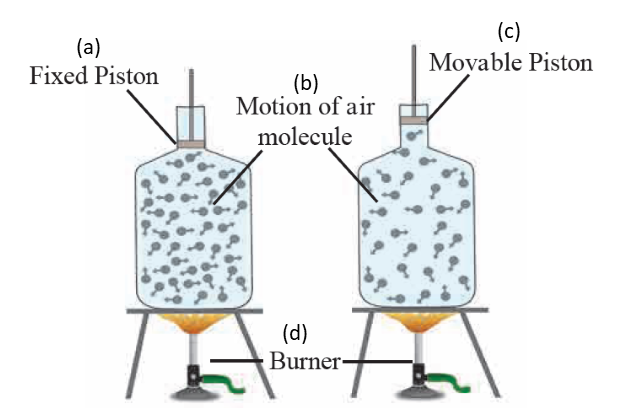3.

 Description °F °C K Freezing point of water ……… 0 ……… Boiling point of water ……… 100 ………
###### Ans
 Description °F °C K Freezing point of water 32 0 273 Boiling point of water 212 100 373.15

# Q. 16. Explain the statement. 9

1. Explain the law in specific heat.

Ans i. The specific heat of an object is the amount of heat required to increase the temperature of unit mass of that substance through one degree.

ii. This is represented by the symbol ‘c’. Its unit in SI is Joule /(kg 0C) and in CGS is cal/(gm 0C).

iii. Suppose Q amount of heat is required to increase the temperature of an object of mass m and specific heat c, from Ti to Tf

iv. This amount depends on the mass and specific heat of the object as well as on the increase in temperature and can be written as.

v. Q = m x c x (Tf – Ti)

2. Explain with the help of formulae the expansion coefficients of liquid and gas.

Ans i. Expansion of liquids

ii. A liquid does not have a definite shape but it has a definite volume.

iii. So we can define a volumetric expansion coefficient for a liquid as follows.

iv. V2 = V1 (1 + b DT) Here, DT is the change in temperature and V1 and V2 are the initial and final volumes of the liquid. b is the volumetric expansion coefficient of the liquid

v. Expansion of gases

vi. A gas does not even have a fixed volume.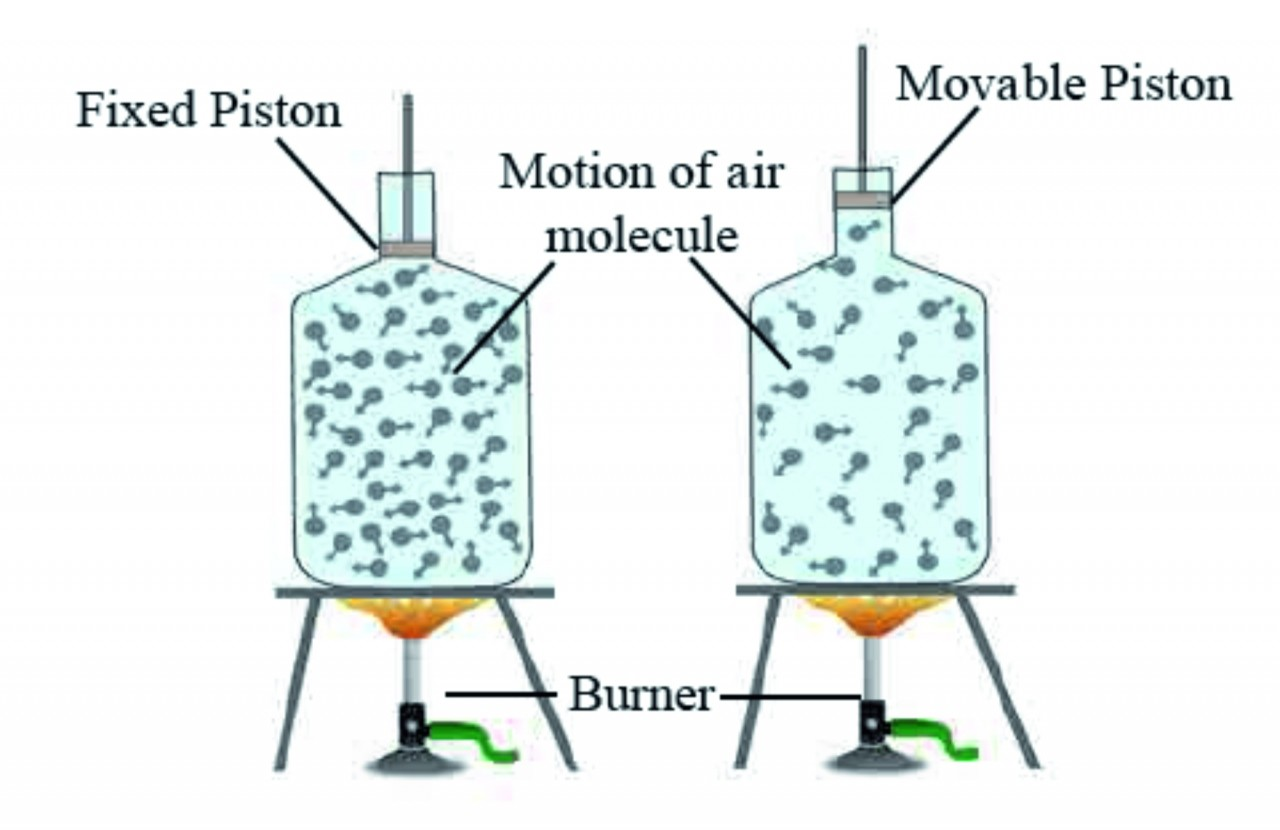vii. Gas expands on heating but if the gas is kept in a closed box, its volume cannot increase but its pressure increases. This is shown in figure given below.

3. State the formula for expansion of solids.

###### Ans

l2 − l1 a l1 × △T

l2 − l1 = λ × l1 × △T l2 = l1 (1+ λ△ T)

λ = (l2 − l1) / (l1 △ T)

1 Thermometers

# Q. 18. Explain with the help of examples : 3

1. Explain the maximum-minimum thermometer with examples of the rmometer?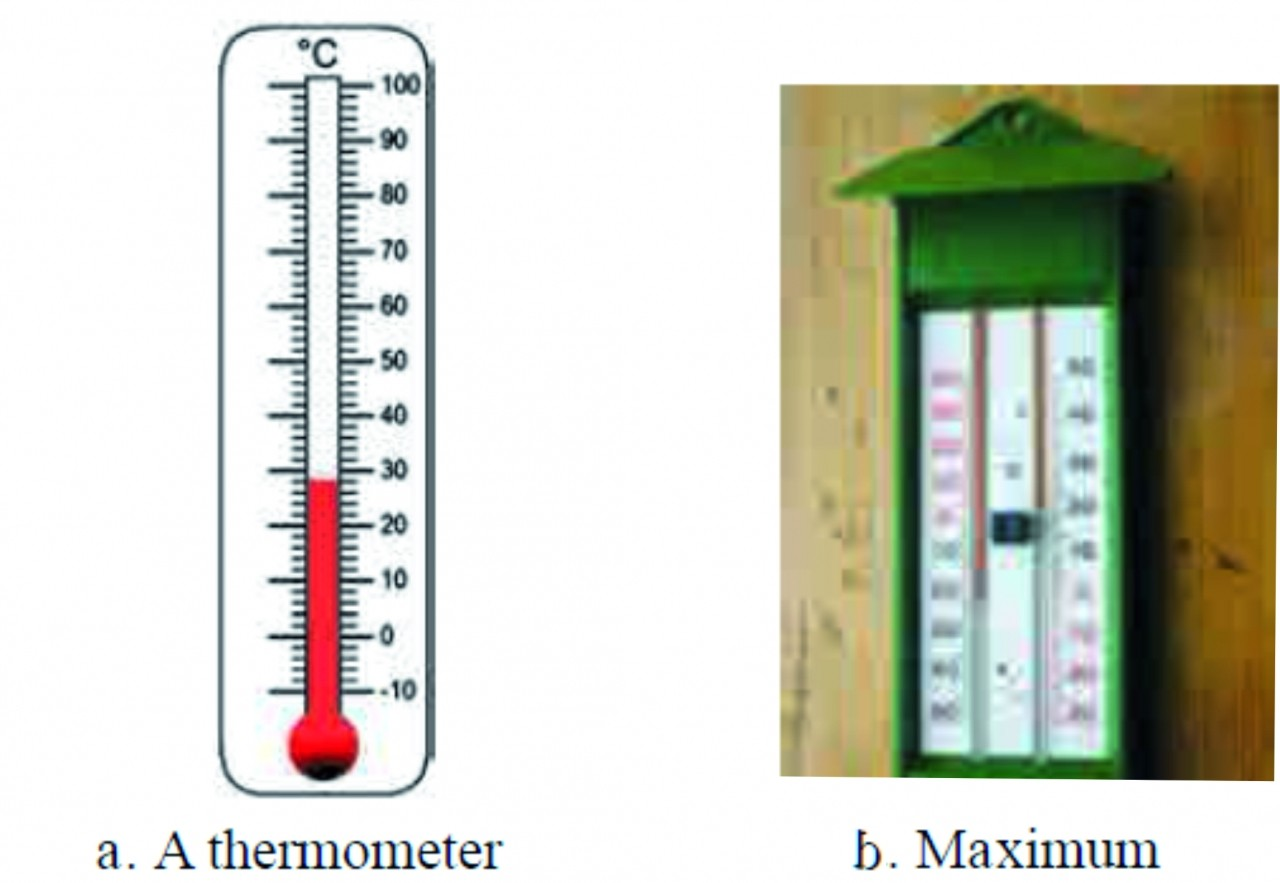Ans The thermometers used in laboratory are similar to the one shown in figure given below as (a) except that the range of temperatures that it can measure is large spanning 40 Celsius to 110 Celsius or even larger. A special type of thermometer is used to measure the minimum and maximum temperatures in a day. It is called the maximum minimum thermometer. It is shown in figure given below as (b)

# Q. 19. Solve Numerical problems 21

1. How much heat will be needed to raise the temperature of 1.5 kg of water from 15ºC to 45ºC ? Give the answer in calories as well as in Joule.

Ans Mass of water = 1.5 kg = 1500 gm.

Change in temperature        = 45ºC – 15ºC

= 30ºC.

Heat required for temperature change = ?

Heat required for temperature change = mass of water (gm) x change in temperature (ºC) cal.

= 1500 × 30 cal

= 45000 cal

= 45000 × 4.18 J

= 188100 J

2. At what temperature will its value be same in Celsius and in Fahrenheit?

Ans If the temperature in Celsius is C, then the temperature in Fahrenheit (F) will be same, i.e. F= C.

(F − 32)/9 = C/5

5 (F – 32)  = 9 C

5F – 160   = 9C

But       C = F

5C – 160 = 9C

-4C = 160

C = -40

C = F = – 40 The temperatures in Celsius and in Fahrenheit will be same at – 40º.

3. Suppose the masses of the calorimeter, the water in it and the hot object made up of copper which is put in the calorimeter are the same. The initial temperature of the calorimeter and water is 30 Celsius and that of the hot object is 60 Celsius . The specific heats of copper and water are 0.09 cal / (gm Celsius) and 1 cal / (gm Celsius) respectively. What will be the final temperature of water?

Ans Given : mw = mo = mc = m, Ti = 30 oC, To = 60 oC, Ti = ?

Using formula

(5), m × (60 − Tf ) × 0.09 = m × (Tf − 30) × 1 + m × (Tf – 30) × 0.09

(60 − Tf ) × 0.09 = (Tf − 30) × 1.09

60 × 0.9 + 30 ´ 1.09 = (0.09 + 1.09) Ti

T1 = 32.29 0C

The final temperature of water will be 32.29 0C.

4. A bridge is made from 20 m long iron rods. At temperature 18°C, the distance between two rods is 0.4 cm. Up to what temperature will the bridge be in good shape?

Ans The bridge would be in good shape until the expansion in the rods does not exceed the gap between them.

Given : Initial length of rod (I1) = 20 m,

Initial temperature (T1) = 18° C,

Final length fo rod (I2) = 20 m + 0.4 cm = (20 + 0.004) m = 20.004 m.

Coefficient of linear expansion for iron (λ iron) = 11.5 × 10-6 (1/°C)

To find : Final temperature (T2)

Formula : I2 = I1 (1 + λ Δ T )

Calculation : From the formula,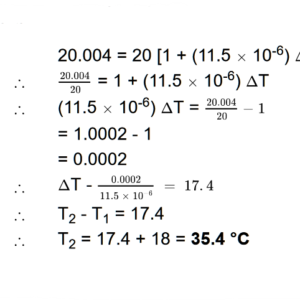The bridge will be in good shape upto the temperature of 35.4 °C.

5. How much will the temperature of 68º F be in Celsius and Kelvin?

Ans Temperature in Fahrenheit = F = 68, Temperature in Celsius = C= ?, Temperature in Kelvin = K = ?

According to formula , (F 32)/5 = C/5

(68 − 32)/5 = C/5

C  =  36/5 × 5

= 20ºC.

K        = C + 273.15

= 20 + 273.15

= 293.15

Thus, the temperature in Celsius = 20 0C and in Kelvin = 293.15 K

6. What must be the temperature in Fahrenheit so that it will be twice its value in Celsius?

Ans Given: F = 2C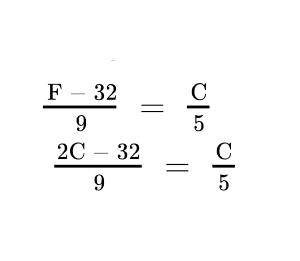∴ (2C – 32) × 5 = C × 9

∴ 10C – 160 = 9C

∴ C = 160°

∴ F = 160 × 2

= 320°

7. If the temperature of water changes by 10ºC on giving 300 cal of heat, what is the mass of water?

Ans Amount of heat given to water = 300 cal,

change in temperature = 10ºC,

mass of water = m = ?

Amount of heat given (cal) = mass of water (gm) x change in temperature (0C)

300 = m x 10

m = 300 / 10 = 30 gm.

# Q. 20. Complete the sentences in paragraph 6

1. Complete the paragraph :

(Temperature, Kinetic energy, Average kinetic, Two, Equal)

The atoms in a substance are always in motion. The total …………… of the atoms in a substance is a measure of the heat contained in that substance, while the …………… of a substance is related to the average kinetic energy of atoms. If the ………… energy of atoms in objects is equal then their…………… will also be …………… .

Ans The atoms in a substance are always in motion. The total Kinetic energy of the atoms in a substance is a measure of the heat contained in that substance, while the Temperature of a substance is related to the average kinetic energy of atoms. If the Average kinetic energy of atoms in Two objects is equal then their Temperature will also be Equal.

2. Complete the paragraph:

###### (Oscillate, Velocities, Atom, Temperatur, Faster, object, Forces )

The ………….. of atoms in a solid object. The in a solid object are tied to one another because of the acting between them. So they cannot be displaced from their places. Because of heat, they………….. around their fixed position. Higher the ………….. of the solid, ………….. is their velocity of oscillation.

Ans The Velocities of atoms in a solid object. The Atoms in a solid object are tied to one another because of the Forces acting between them. So they cannot be displaced from their places. Because of heat, they Oscillate around their fixed position. Higher the Temperature of the solid Faster is their velocity of oscillation.

# Q. 21. Answer the following 15

1. Describe a clinical thermometer. How does it differ from the thermometer used in laboratory?

Ans i. A clinical thermometer consists of a narrow glass tube having a bulb at one end.

ii.The part of the tube along with the bulb is filled with mercury or alcohol. As mercury is harmful to health, alcohol is preferred.

iii.The rest of the tube is evacuated and the other end of the tube is closed.

iv.The bulb is kept in contact with the body of a person whose body temperature is to be measured. Thus, the temperature of the bulb becomes same as that of the body of the person.

v.If the body temperature is high, because of the increased temperature, the alcohol inside the tube expands and it rises in the tube. The body temperature can be obtained from the level of the alcohol inside the tube.

Vi. As the clinical thermometer is mainly used to measure body temperature, the range of this thermometer is marked from 35 C to 42 C.

vii. The thermometer which is used in laboratories is constructed in similar manner except that the thermometers in laboratory can measure temperatures ranging from 40 C to 110 C or larger in some cases.

2. Explain the construction of a calorimeter. Draw the necessary figure.

Ans A calorimeter is a device used to measure heat content of an object.

###### Construction:

i. A calorimeter consists of two vessels similar to that of thermos flask. The inner vessel is made of copper.

iii. The space between inner vessel and the outer vessel is filled with insulator like air. Both the vessels are connected to each other by a heat resistant ring so that, no heat is transferred between the inner vessel and the surroundings. Thus, the inner vessels is thermally isolated from the surroundings.

iii. Both the vessels have a single heat resistant lid fitted on top. There are two holes made on the lid from which a thermometer and a stirrer are inserted in the inner vessel.

iv. Thermometer is used to record the temperature of the liquid and stirrer is used for stirring the liquid.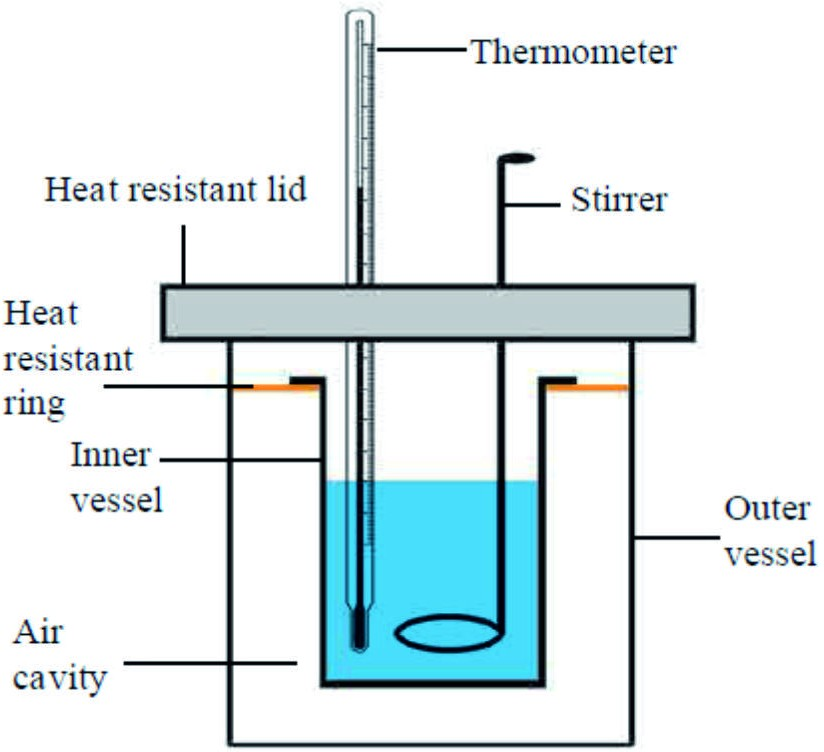3. Explain why rails have gaps at specific distances.

Ans i. During summer, due to change in temperature, the length of the rails increases.

ii. If the rails are made without the gaps in between, then after expansion, the rails may get distorted in summer, leading to rail accidents.

iii. Hence, to accommodate this change in length of the rails, they have gaps at specific distances.

4. Nishigandha kept a vessel containing all the ingredients for making tea in a solar cooker. Shivani kept a similar vessel on a stove. Whose tea will be ready first and why?

Ans i. Solar cooker uses heat energy generated in the Sun.

ii. The Sun being far away from the earth, very small amount of heat reaches the earth, making the process of heating slow and time-consuming.

iii. Whereas, the stove converts the chemical energy stored in fuels into heat energy.

iv. This energy generated is transferred to vessel in large amounts. Hence, the tea made by Shivani using a stove will be ready first.

5. Explain with the help of formulae the expansion coefficients of liquid and gas.

###### Ans: Coefficient of expansion for liquids:

i.Liquids do not have definite shapes or sizes, but they have a definite volume. Hence, liquids possess a volumetric expansion coefficient.

ii. Let liquid of initial volume V1 expands to volume V2 when heated. If ΔT is the change in temperature, then, V2 = V1 (1 + βΔT)

Here, is volumetric expansion coefficient of the liquid.

###### Coefficient of expansion for gases:

i. Gases do not possess definite shape, size or volume. They occupy the volume of the container.

ii. Gases expand on heating when kept in a open container. If the gas is kept in a closed container and heated, then the pressure on the gas increases while, the volume remains unchanged.

iii. Thus, the expansion of a gas is measured under constant pressure and the expansion coefficient is termed as constant pressure expansion coefficient.

iv. If a gas is heated in a beaker with a movable piston attached to the beaker, then pressure remains constant while volume increases. In such a case, volumetric expansion is given as,

V2 = V1 (1 + βΔT)

Here, V2 V1

β

= Final volume of the gas,

= Initial volume of the gas,

= Constant pressure expansion coefficient,

ΔT = Change in the temperature.

# Q. 22. Draw a well labeled diagram and explain. 5

1. Explain heat and temperature with the diagram of Motion of atoms in gas and solid.

Ans Motion of atoms in gas and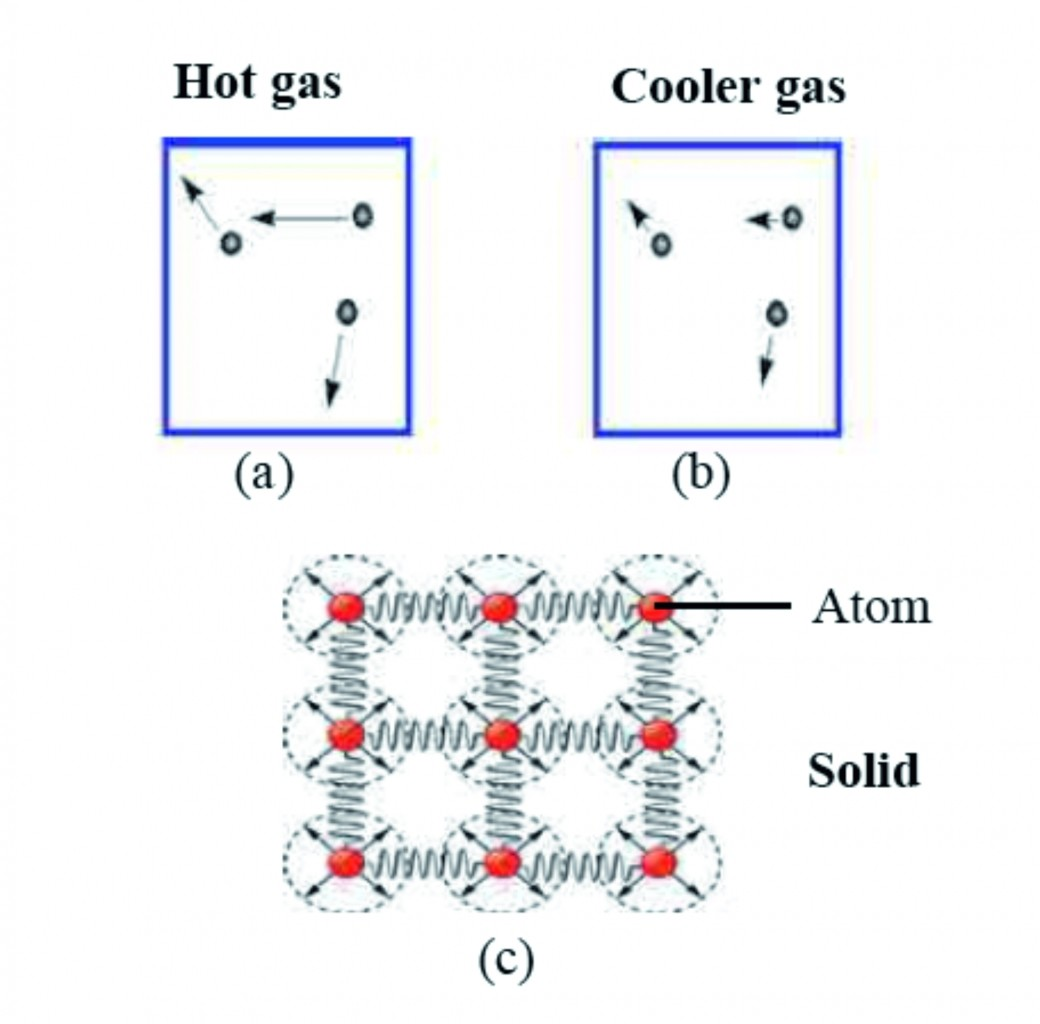###### Diagram : Motion of atoms in gas and solid

i. We know that a substance is made of atoms.

ii. The atoms in a substance are always in motion.

iii. The total kinetic energy of the atoms in a substance is a measure of the heat contained in that substance, while the temperature of a substance is related to the average kinetic energy of atoms.

iv. If the average kinetic energy of atoms in two objects is equal then their temperatures will also be equal.

v. Figure given above ‘a’ and ‘b’ show the velocities of atoms in a gas at high and low temperature, respectively.

vi. The direction and the length of the arrows attached to the atoms show the direction and magnitude of the velocity of the atoms.

vii. The velocity of atoms in the gas at higher temperature is larger than the velocity of atoms in the gas at lower temperature.

# Q. 23. Answer the following in detail 5

1) Label the calorimeter2) Enlist the part of calorimeter and explain.

Ans i. A calorimeter similar to a thermos flask, a calorimeter has two vessels, an inner and an outer one.

ii. This way, no heat can be transferred from the inner to the outer vessel or from the outer to the inner vessel.

iii. Thus, the inner vessel is thermally isolated from the surroundings.

iv. The inner vessel is made of copper.

v. A thermometer for measuring the temperature and a stirrer for stirring the liquid in the calorimeter are fitted in it.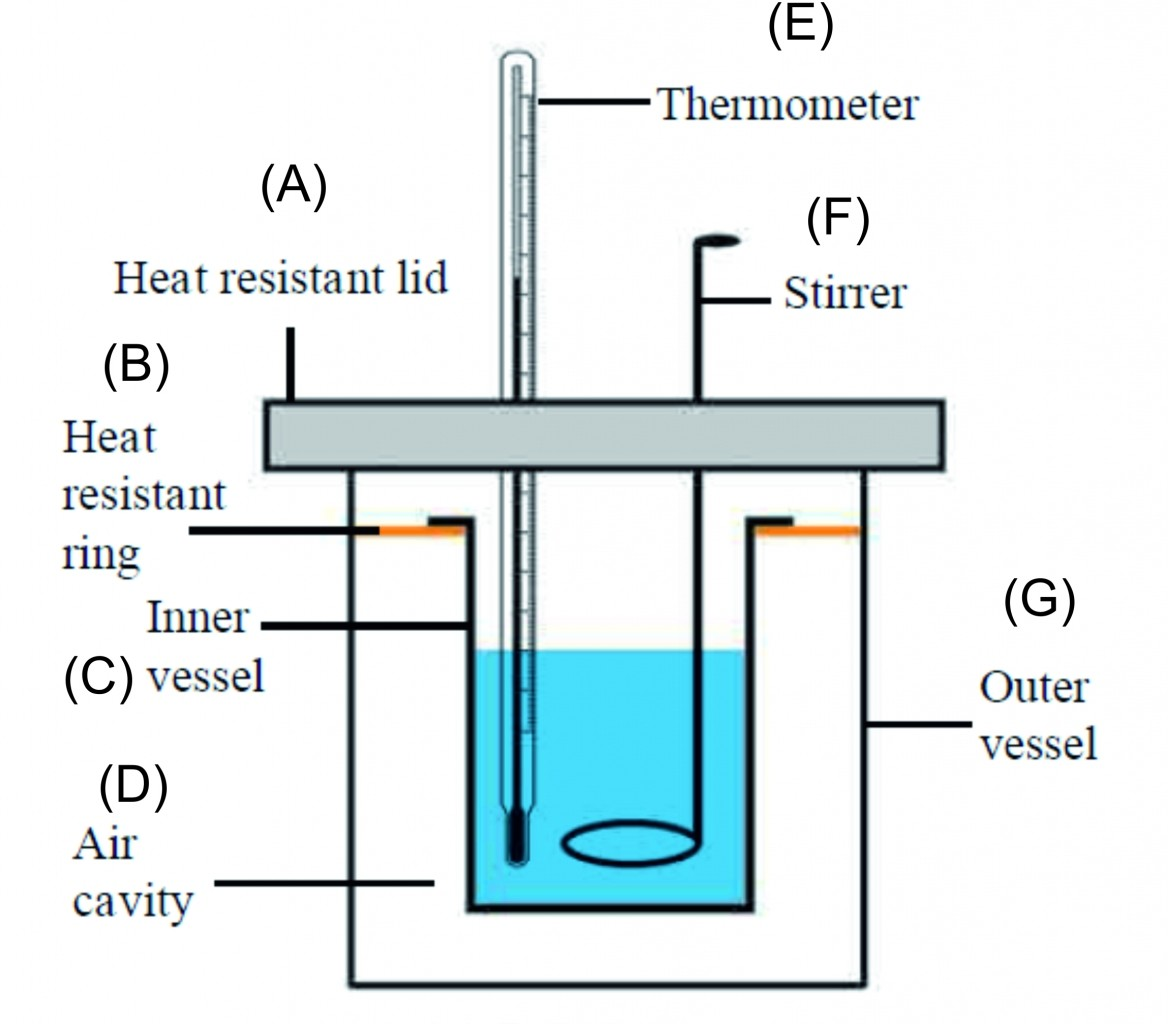vi.

This site uses Akismet to reduce spam. Learn how your comment data is processed.